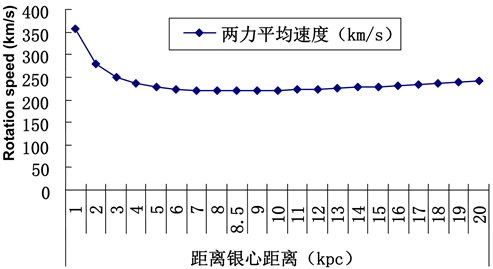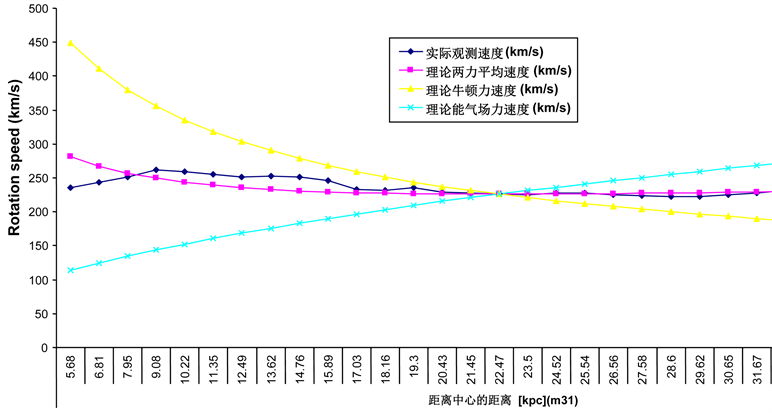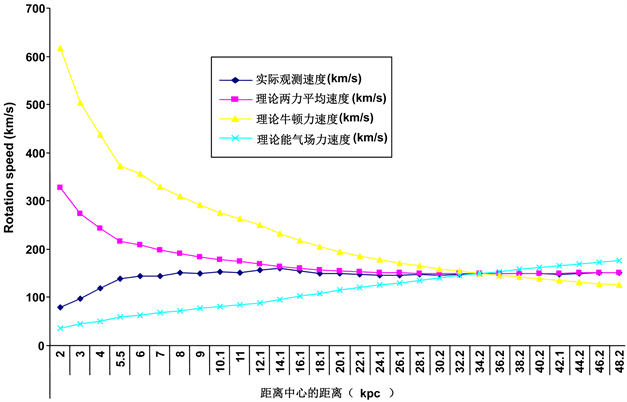# 物质–暗物质–暗能量三位一体Matter-Dark Matter-Dark Energy Are a Whole

DOI: 10.12677/MP.2018.85026, PDF, HTML, XML, 下载: 800  浏览: 2,463

Abstract: Space-time ladder theory reveals that the space-time of Qi is the origin of the universe. The polarization Qi space-time produces physical space-time and metaphysical space-time. Physical space-time is matter, metaphysical space-time is dark energy, and Qi space-time is dark matter. So matter, dark matter and dark energy are a whole. The polarization Qi space-time generates an energy Qi field, and the energy Qi field contains an energy field and a Qi field. Based on the energy field, we calculate the theoretical values of the rotation curves of the three galaxies. These theoretical values are basically consistent with the actual observations. Based on the Qi field theory, we use the Hubble constant as the corresponding value of the Qi field strength, and calculate the theoretical value of the anomalous acceleration of the pioneer, which is basically consistent with the previous actual calculation. The key here is that the calculation of dark matter and dark energy comes from the same theory, that is, the theory of energy Qi field and the theoretical values of both are basically consistent with the actual observations, which proves that the theory of energy Qi field is correct.

1. 引言

${v}_{1}={H}_{0}D$

${v}_{2}=\frac{RQ}{\mathrm{sin}\theta }$

2. 暗物质问题的有关计算

1) 能量场是由能量生成。能量线开始于能量收缩态，终止于能量膨胀态。能量收缩态就是原子核状态，能量膨胀态就是暗能量。

2) 气场线没有初始点，也没有终止点。气场线会形成循环或延伸至无穷远。换句话说，进入任何区域的气场线，也必须从那区域离开。通过任意闭曲面的气通量等于零，气场是一个螺线矢量场。

3) 描述随时间变化的气场生成能量场。

4) 气场可以用两种方法生成：一种是靠能量流产生，另一种是靠随时间变化的能量场产生。

${F}_{1}=q\left({E}_{1}+{v}_{1}×B\right)$

$F=m\left(E+v×Q\right)$ ，(可以称之为洛伦兹力能气方程)。

$R=\frac{mv\mathrm{sin}\theta }{Bq}$

$T=\frac{2\text{π}m}{Bq}$

$h=\frac{2\text{π}mv\mathrm{cos}\theta }{Bq}$

$R=\frac{mv\mathrm{sin}\theta }{Qm}=\frac{v\mathrm{sin}\theta }{Q}$

$T=\frac{2\pi m}{Qm}=\frac{2\text{π}}{Q}$

$h=\frac{2\text{π}mv\mathrm{cos}\theta }{Qm}=\frac{2\text{π}v\mathrm{cos}\theta }{Q}$

$F=\frac{m{v}^{2}}{R}=\frac{GMm}{{R}^{2}}$

$v=\sqrt{\frac{GM}{R}}$

$v=\frac{RQ}{\mathrm{sin}\theta }$

$v=\sqrt{\frac{GM}{R}}=\frac{RQ}{\mathrm{sin}\theta }$

$\frac{GM}{R}=\frac{{R}^{2}{Q}^{2}}{{\left(\mathrm{sin}\theta \right)}^{2}}$

$T=\frac{2\text{π}m}{Qm}=\frac{2\text{π}}{Q}$

$\frac{GM}{R}=\frac{{R}^{2}{Q}^{2}}{{\left(\mathrm{sin}\theta \right)}^{2}}=\frac{4{\text{π}}^{2}{R}^{2}}{{\left(\mathrm{sin}\theta \right)}^{2}{T}^{2}}$

$\frac{{T}^{2}}{{R}^{3}}=\frac{4{\text{π}}^{2}}{GM{\left(\mathrm{sin}\theta \right)}^{2}}$

$v=\frac{RQ}{\mathrm{sin}\theta }$$v$ 是星体的运动速度， $R$ 是星体距离核心的距离， $Q$ 是气感应强度(或者气场强度)。电场强度

1) 银河系自转曲线的理论计算与实际观测比较Table 1. Star speeds caused by Newton’s gravitational, energy Qi field and resultant forces (Milky Way)Figure 1. The theoretical rotation curve of the milky way

1983年 ，Schneider和Terzian 利用Schneider等人 所提供的524个PN，对其中有距离测定值的250个PN做了一项研究，样本银心距范围4 < R < 19 kpc。他们发现V太阳 ≈ 220 km/s，而当R > 8.5 kpc时自转曲线有所抬高；不过，远银心距PN转动速度的不确 定性相当大(参见文献 之图2)。后来的一些研究表明，自R = 8.5 kpc起外盘的自转曲线基本上是平坦的，V (R)值并未表现出有明显增大或减小的趋势 ；这些工作所用的样本PN，尽管个数有的已接近900 ，但银心距均不超过14 kpc。

2) 仙女座星系(M31)自转曲线的理论计算与实际观测比较

m/s2，我们用这个相对固定的数值，计算能气场力的星体速度 $v=\sqrt{RE}$ ，同时我们计算牛顿引力的速度

$v=\sqrt{\frac{GM}{R}}$Table 2. Comparison of actual observation speed with theoretical calculation (Andromeda Galaxy)Figure 2. Comparison rotation curve between actual observation and theoretical calculation (M31)

3) NGC 3198星系自转曲线的理论计算与实际观测比较

34.2 kpc以内的质量，这个统一的NGC 3198星系的质量，将影响计算牛顿引力的速度 $v=\sqrt{\frac{GM}{R}}$ ，也就

3. 暗能量问题的有关计算

$v={H}_{0}D$ (1)Table 3. Comparison of actual observation speed with theoretical calculationFigure 3. Comparison rotation curve between actual observation and theoretical calculation (NGC 3198)

=(299792458 m/s × 67740 m/s)/3.086 e + 22 m

=6.58066788882696 × 1010 m/s2

=(299792458 m/s × 73450 m/s)/3.086 e + 22 m

=7.13537136749837977965 × 10−10 m/s2

6.858019628162669889825 × 10−10 m/s2

1.8463112134 × 10−10 m/s2 + 6.858019628162669889825 × 10−10 m/s2

=8.704330841562669889825 × 10−10 m/s2。这个是先驱者号的能气场力的加速度，应该算是理论预测值。

$F=\frac{m{c}^{2}}{r}\frac{{c}^{n}}{{v}_{1-n!}}$ ，其中， $\frac{{c}^{n}}{{v}_{1-n!}}$ 是时空阶梯因子，这个时空阶梯因子，让力从非常弱的牛顿引力一直到非常强的强力。

4. 暗物质和暗能量问题的综合考虑

5. 总结

  常炳功. 时空阶梯的建立以及对双缝实验的解释[J]. 科技展望, 2016(1): 216-220, 222.  常炳功. 暗物质是能量场气场物质, 类似电场磁场物质[J]. 现代物理, 2018, 8(3): 65-73.  赵君亮. 星系自转曲线之观测研究进展[J]. 天文学进展, 2013, 31(2): 125-140.  常炳功. 能量与中医气的关系类似电与磁的关系[J]. 现代物理, 2018, 8(2): 27-34.  McHutchon, A. (2013) Electromagnetism Laws and Equations. Michaelmas.  开普勒定律, 维基百科, 自由的百科全书.  Walker, M.G. and Peñarrubia, J. (2011) A Method for Measuring (Slopes of) the Mass Profiles of Dwarf Spheroidal Galaxies. The Astrophysical Journal, 742, 1-20. https://doi.org/10.1088/0004-637X/742/1/20  Rubin, V.C., Ford, W.K. and Thonnard, N. (1977) Extended Rotation Curves of High-Luminosity Spiral Galaxies. I—The Angle between the Rotation Axis of the Nucleus and the Outer Disk of NGC 3672. Astrophysical Journal, 217, L1-L4. https://doi.org/10.1086/182526  Rubin, V.C., Ford, W.K. and Thonnard, N. (1978) Extended Rotation Curves of High-Luminosity Spiral Galaxies. IV—Systematic Dynamical Properties, SA through SC. Astrophysical Journal, 225, L107-L111. https://doi.org/10.1086/182804  Rubin, V.C. (1979) Rotation Curves of High-Luminosity Spiral Galaxies and the Rotation Curve of Our Galaxy. The Large-Scale Characteristics of the Galaxy, 84, 211-220. https://doi.org/10.1007/978-94-009-9503-1_38  Moffat, A.F.J. (1993) Precision Photometry of Young Stellar Groups to-wards the Outer Galactic Disk and the Galactic Rotation Curve. Astronomical Journal, 105, 1831-1854. https://doi.org/10.1086/116558  Blitz, L. (1979) The Rotation Curve of the Galaxy to R = 16 Kiloparsecs. Astrophysical Journal, 231, L115-L119. https://doi.org/10.1086/183016  Jackson, P.D., FitzGerald, M.P. and Moffat, A.F.J. (1979) Recent Evidence on the Rotation Curve of Our Galaxy for R > Ro. IAUS, 84, 221.  Merrifield, M.R. (1992) The Rotation Curve of the Milky Way to 2.5 R0 from the Thickness of the H I Layer. Astronomical Journal, 103, 1552-1563. https://doi.org/10.1086/116168  Honma, M. and Sofue, Y. (1997) Rotation Curve of the Galaxy. Publications of the Astronomical Society of Japan, 49, 453-460. https://doi.org/10.1093/pasj/49.4.453  Schneider, S.E. and Terzian, Y. (1983) Planetary Nebulae and the Galactic Rotation Curve. Astrophysical Journal, 274, L61-L64. https://doi.org/10.1086/184151  Schneider, S.E., Terzian, Y., Purgathofer, A., et al. (1983) Radial Velocities of Planetary Nebulae. Astrophysical Journal Supplement Series, 52, 399-423. https://doi.org/10.1086/190874  Maciel, W.J. and Dutra, C.M. (1992) Kinematics of Disk Planetary Nebulae. Astronomy and Astrophysics, 262, 271-280.  Koupelis, T. and Kuhn, K.F. (2007) Quest of the Universe. Jones & Bartlett Publishers, Burlington, 492.  Chemin, L., Huchtmeier, W.K. and Lockman, F.J. (2006) Extended HI Rotation Curve and Mass Distribution of M31. The Astrophysical Journal, 641, L109-L112.  Karukes, E.V., Salucci, P. and Gentile, G. (2015) The Dark Matter Distribution in the Spiral NGC 3198 out to 0.22 Rvir. Astronomy & Astrophysics, 578, A13.  Gomez-Valenta, A. and Amendolab, L. (2018) H0 from Cosmic Chronometers and Type Ia Supernovae, with Gaussian Processes and the Novel Weighted Polynomial Regression Method.  Lammerzahl, C., Preuss, O. and Dittus, H. (2006) Is the Physics within the Solar System Really Unders-tood?  Anderson, J.D, Laing, P.A., Lau, E.L., Liu, A.S., Nieto, M.M. and Turyshev, S.G. (1998) Indication, from Pioneer 10/11, Galileo, and Ulysses Data, of an Apparent Anomalous, Weak, Long-Range Acceleration. Physical Review Letters, 81, 2858-2861. https://doi.org/10.1103/PhysRevLett.81.2858  Anderson, J.D., Laing, P.A., Lau, E.L., Liu, A.S., Nieto, M.M. and Turyshev, S.G. (2002) Study of the Anomalous Acceleration of Pioneer 10 and 11. Physical Review D, 65, Article ID: 082004. https://doi.org/10.1103/PhysRevD.65.082004  常炳功. 时空阶梯理论的历史以及封顶问题[J]. 现代物理, 2016, 6(4): 136-147.  Akinto, O.F. and Tahir, F. (2016) Strong Gravity Approach to QCD and General Relativity. arXiv:1606.06963v3 [physics.gen-ph]31. https://arxiv.org/pdf/1606.06963.pdf